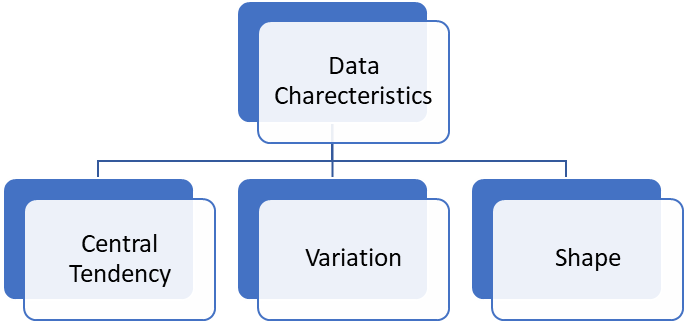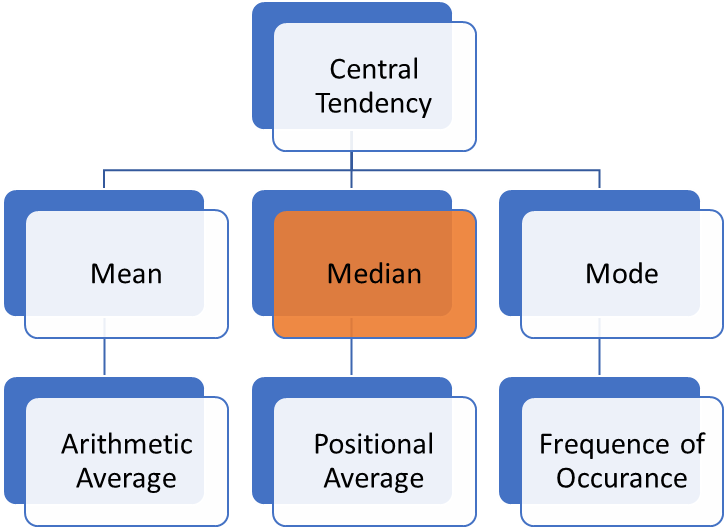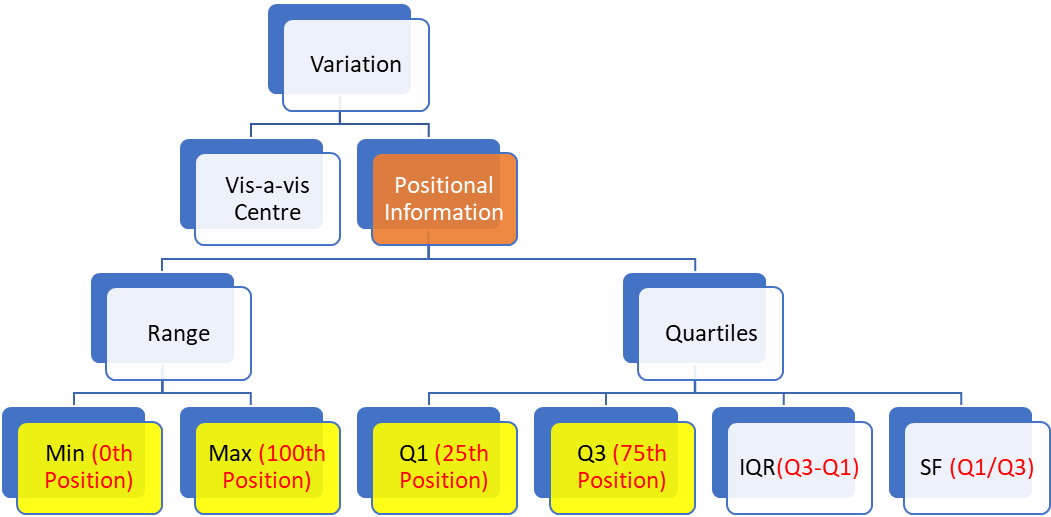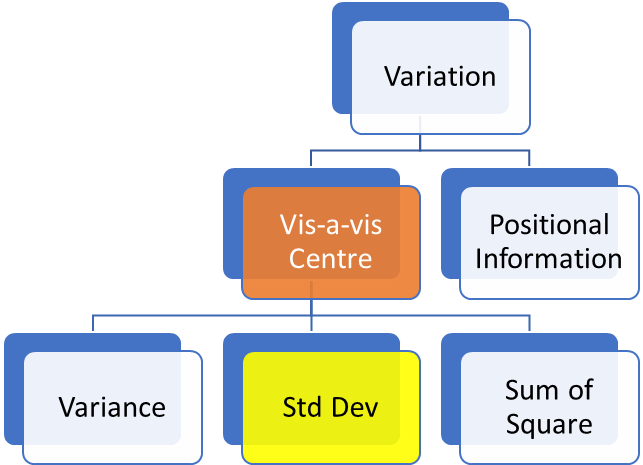# Lean Six Sigma - Basic Statistics Session

Why need Data?

• To analyse performance
• To understand the system
• To review the system
• To take the correct decision
• To conclude results
• For Comparison
• Identify deviations
• Future Planning
• For prediction or anticipation
• Find out discrepancies
• Comparison with std
• Determine the cause of the problem
• Review of plans and Goals
• Eliminate repeatability
• Visualize relationshipWhat do we need Data?

• Analyze the current situation
• To Analyze trend
• Prepare for corrective action
• Predict

# Central Tendency

Data tends to be close to its centre

CT = 15 Km/Litre

Milege of car# Mean

Is a good measure of central tendency when there is not too much variation in data.

Arithmetic average

Disadvantage – Gets impacted by extreme high or extreme low values

10 Families – 1000 pounds = 10000

Avg = sum of all/ number of all observations

= 1000 + 1000 + 1000 + 1000 + 1000 1000 + 1000 + 1000 + 1000 + 1000 /10

Average or Mean = 1000 pounds

LNM =46.5Bn Pounds

Avg = 1000 + 1000 + 1000 + 1000 + 1000 1000 + 1000 + 1000 + 1000 + 1000 + 46.5 Bn /11

= 4.6 Bn Pounds

This locality has billionaires only

150 – 194, 28000

Median

• Prefer median when your data has high variation
• data is arranged in Ascending order. And the 50th position becomes your median.
• Advantage – Does not get impacted by extreme high or low data points

• Disadvantage – Because it is positional value, it can’t be used for mathematical calculations

Avg = 1L  Total = 1L* 11 = 11L

Median = 1L = 50% time value is less than equal to 1L,

Recruiters – 35K * 20 = 7 L

Median = 27K –

# Mode –

Should be used only when you have limited possibilities

Frequency of occurrence

Batsman =

0 1 2 3 4 5 6

 1 2 3 4 5 6 7 12 14 19 100 2 100 1

Which data is occurring the most

Mode = 0

Mode = Dice

 1 2 3 4 5 6 12 14 19 85 2 42

Mode = 4

 1 2 3 4 5 6 12 14 92 92 2 25

Mode =3,4 – referred as bi-modal

 1 2 3 4 5 6 12 14 25 25 25 2

Mode = 3,4,5 – Tri Modal

# VariationRange = Max - Min

Quartiles will always have 25% data

Min – Q1 = 25%

Q1 – Median (Q2) = 25%

Q2 – Q3 =

IQR – Inter Quartile Data = Q3- Q1

SF = Q1/Q3 best case = 1, worst = as far away as possible

Range = Max – Min1 2 3 4 5 = 15/5 = 3

Distance of data from Centre

Mean = 3

(1-3) + (2-3) + (3-3) + (4-3) + (5-3) =

Mean = 1 + 2 + 3 + 4 + 5/5 = 15/5 = 3

(1-3) + (2-3) + (3-3) + (4-3) + (5-3) = (-2) + (-1) + (0) + (+1) + (+2) = 0

(1-3)^2 + (2-3) ^2+ (3-3)^2 + (4-3)^2 + (5-3)^2 = 10

4+ 1+0+1+4= 10

1,2,3,4,5,3 -

Sum of Square of distance of data from centre = SS

Avg of Sum of Square of distance of data from centre = Variance

(1-3)^2 + (2-3) ^2+ (3-3)^2 + (4-3)^2 + (5-3)^2 / n-1

1 2 3 4 5

Mean = 3

(1-3) + (2-3) + (3-3) + (4-3) + (5-3) = 0

(1-3)^2 + (2-3) ^2  + (3-3) ^2 + (4-3) ^2  + (5-3) ^2 = Sum of Square

4 + 1 + 0 + 1 + 4 = 10 = Sum of Square of data from its centre (mean)

(1-3)^2 + (2-3) ^2  + (3-3) ^2 + (4-3) ^2  + (5-3) ^2  / n-1 = Variance =average of Sum of Square of data from its centre (mean)

Variance = 10/4 = 2.5

(1-3)^2 + (2-3)^2 + (3-3)^2 + (4-3)^2 + (5-3)^2 =  Sum of Square

Average of Sum of Square = Variance

Sqr Root of Variance = Std Dev

1,2,3,4,5

Mean =3

(1-3)+ (2-3) + (3-3) + (4-3) + (5-3) = 0

(1-3)^2+ (2-3)^2 + (3-3)^2 + (4-3)^2 + (5-3)^2

4 + 1+ 0 + 1 + 4 = 10 =>> Sum of Square

Variance = Sum of Square/ n-1

Std Dev = Sqr root of Variance

Variation

Mean = 3

(1-3)^2+(2-3) ^2+(3-3) ^2+(4-3) ^2+(5-3) ^2 =

+ 4 + 1 + 0 + 1 + 4 = 10 = Sum of Square >> This is squared sum of distance of data from its centre

SS/n-1 = 10/4 = 2.5 = Variance (Avg sum of Square) >> avergage of squared sum of distance of data from its centre

Variance Sqr Root = Std Dev

(1-3)^2+(2-3)^2+(3-3)^2+(4-3)^2+(5-3)^2 = Sum of Square

= Sum of Squared distance of data from its centre

(1-3)^2+(2-3)^2+(3-3)^2+(4-3)^2+(5-3)^2 / n-1 = Variance

Std Dev = Srq Root of Variance

# Normality

If a process is to be considered “Normal” it will follow the below rules

• Mean + 1 Std Dev Mean – 1 Std Dev = 68%
• Mean + 2 Std Dev Mean – 2 Std Dev = 95%
• Mean + 3 Std Dev – 3 Std Dev = 99.73%

The Process is considered non normal or out of control and the reason is considered special cause variation. Statistics mandates that u must conduct RCA for such special behaviour.

Mileage----  Mean = 15 Std Dev = 1

 Lower Upper Mileage 68% 15 -1=14 15+1=16 14-16 95% 15-2(1) 15+2(1) 13-17 99.73% 15-3(1) 15+3(1) 12-18

22 ---

Production

Mean = 200 Std Dev = 20

68% à

 68% 200 + 20 200 – 20 180 - 220 95% 200 + 2(20) 200 – 2(20) 160 - 240 99.73 200 +3(20) 200 – 3(20) 140 - 260

Under normal conditions the production will be 140 – 260 (we are 99.73% sure)

Target - 300

Route1:           Mean = 30 Min                Std Dev = 4 Min

Route 2:          Mean = 20 Min               Std Dev = 20 Min

 Route1 68% 95% 99.73% 30 + 3(4) =30 + 12 30 – 3(4)= 30 – 12 = 18 18  - 42 Min

 Route2 68% 95% 99.73% 20 + 3(20)= 20 + 60 = 80 20 – 3(20) = 0 0 – 80 Min

HDFC  Avg =>10 Std Dev =1 from 7% to 13 %

Mean + 3 Std Dev = 10 + 3 = 13

Mean – 3 Std Dev = 10 – 3 = 7

ICICI => Mean =20 Std Dev = 20 Return -40% to 80%

Mean + 3 Std Dev = 20 + 3(20) = 20 + 60 = 80

Mean – 3 Std Dev = 20 – 60 = -40%

Milege

Mean = 20 Std Dev 1

68 % -> 21 – 19

95% -à 22 -18

99.73% --à 23 – 17

Under normal circumstance this is what my performance will be

Everytime you see special behaviour – you must conduct RCA

Consider that process is “normal” or within control

Even if one data is outside – we term that as special

Mean = 25 Min, Std Dev = 1

26 -- 24

Mean + 2 Std Dev Mean – 2 Std Dev = 95%

27 Min- 23 Min = >> 95%

Mean + 3 Std Dev – 3 Std Dev = 99.73%

28 -- 22

# Normality

Normal

Statistics has a definition of the term “normal”

Following criteria:

• Mean +- 1 Std Dev = 68% of data

13-17

• Mean + _ 2 Std Dev = 95%

11 – 19 Milege

• Mean +- 3 Std Dev = 99.74 % of data

15 +6 15-6 = 21----9

If any data adheres to the above, it is referred to as normal (or following normal distribution)

If any of the above is not met – process is considered non normal and there is presence of special cause variation in data.

Milege

Mean = 15

Std Dev = 1

16 – 14 – 68% - it is 68% likely that milege shall be between 14 to 16

13 to 17 – 95% - You are 95% sure that milege shall be between 13 – 17

12-18 – 99.74

Mean = 25 Min

Std Dev = 2 Min

25 + 2 = 27

25-2 = 23

23  --- 27 = 68% time

HDFC MF = mean = 10%                  Std Dev = 1

ICICI MF = Mean 40 %                      Std Dev = 20%

 68% 95% 99.73 % HDFC 9-11 8-12 7-13 ICICI 20-60 0-80 -20 - 100

Normal Data exhibits these properties:

• Mean Median Mode will be equal
• Unimodal i.e. you will have only one mode and that shall be at the centre
• Bell curve will accommodate entire process performance
• If you divide the bell curve at the centre, you will always have identical behaviour both sides.
• Bell curve never touches the axis

Mean must be used with Std Dev ( never use mean alone)

Median should be used with Min Q1 Median Q3 Max

25 min

Std dev =2 min

99.74%

25 + (3*2) = 31

25 – (3*2) = 19

30 min Target

95%

25 + 4 = 29

25-4  = 21

Std Deviation

Variation

• Vis-à-vis Centre
• Centre = Mean, we want to study distance of data from centre
• 1,2,3,4,5
• Mean = 3
• Distance from Centre
• (1-3) + (2 -3) + (3-3) + (4-3) + (5-3) = 0
• Because of this challenge – they squared the same
• (1-3)^2+ (2 -3)^2 + (3-3)^2 + (4-3)^2 + (5-3)^2
• Sum of Square is the sum of squared data from its mean
• Variance = Avg = (1-3)^2+ (2 -3)^2 + (3-3)^2 + (4-3)^2 + (5-3)^2 / n-1
• Sum of Square = 10/4 = 2.5
• Std Dev = Sqr root of Variance
• Root of the avg distance of data from its centre
• Positional Information
• Quartiles
• Q1
• Q3
• IQR
• SF
• Range
• Min
• Max